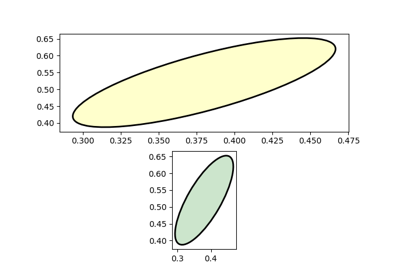# matplotlib.patches.Arc¶

class `matplotlib.patches.``Arc`(xy, width, height, angle=0.0, theta1=0.0, theta2=360.0, **kwargs)

An elliptical arc. Because it performs various optimizations, it can not be filled.

The arc must be used in an `Axes` instance—it can not be added directly to a `Figure`—because it is optimized to only render the segments that are inside the axes bounding box with high resolution.

The following args are supported:

xy
center of ellipse
width
length of horizontal axis
height
length of vertical axis
angle
rotation in degrees (anti-clockwise)
theta1
starting angle of the arc in degrees
theta2
ending angle of the arc in degrees

If theta1 and theta2 are not provided, the arc will form a complete ellipse.

Valid kwargs are:

Property Description
`agg_filter` a filter function, which takes a (m, n, 3) float array and a dpi value, and returns a (m, n, 3) array
`alpha` float or None
`animated` bool
`antialiased` or aa bool or None
`capstyle` [‘butt’ | ‘round’ | ‘projecting’]
`clip_box` a `Bbox` instance
`clip_on` bool
`clip_path` [(`Path`, `Transform`) | `Patch` | None]
`color` matplotlib color spec
`contains` a callable function
`edgecolor` or ec mpl color spec, None, ‘none’, or ‘auto’
`facecolor` or fc mpl color spec, or None for default, or ‘none’ for no color
`figure` a `Figure` instance
`fill` bool
`gid` an id string
`hatch` [‘/’ | ‘' | ‘|’ | ‘-‘ | ‘+’ | ‘x’ | ‘o’ | ‘O’ | ‘.’ | ‘*’]
`joinstyle` [‘miter’ | ‘round’ | ‘bevel’]
`label` object
`linestyle` or ls [‘solid’ | ‘dashed’, ‘dashdot’, ‘dotted’ | (offset, on-off-dash-seq) | `'-'` | `'--'` | `'-.'` | `':'` | `'None'` | `' '` | `''`]
`linewidth` or lw float or None for default
`path_effects` `AbstractPathEffect`
`picker` [None | bool | float | callable]
`rasterized` bool or None
`sketch_params` (scale: float, length: float, randomness: float)
`snap` bool or None
`transform` `Transform`
`url` a url string
`visible` bool
`zorder` float
`draw`(renderer)

Ellipses are normally drawn using an approximation that uses eight cubic bezier splines. The error of this approximation is 1.89818e-6, according to this unverified source:

Lancaster, Don. Approximating a Circle or an Ellipse Using Four Bezier Cubic Splines.

http://www.tinaja.com/glib/ellipse4.pdf

There is a use case where very large ellipses must be drawn with very high accuracy, and it is too expensive to render the entire ellipse with enough segments (either splines or line segments). Therefore, in the case where either radius of the ellipse is large enough that the error of the spline approximation will be visible (greater than one pixel offset from the ideal), a different technique is used.

In that case, only the visible parts of the ellipse are drawn, with each visible arc using a fixed number of spline segments (8). The algorithm proceeds as follows:

1. The points where the ellipse intersects the axes bounding box are located. (This is done be performing an inverse transformation on the axes bbox such that it is relative to the unit circle – this makes the intersection calculation much easier than doing rotated ellipse intersection directly).

This uses the “line intersecting a circle” algorithm from:

Vince, John. Geometry for Computer Graphics: Formulae, Examples & Proofs. London: Springer-Verlag, 2005.

2. The angles of each of the intersection points are calculated.

3. Proceeding counterclockwise starting in the positive x-direction, each of the visible arc-segments between the pairs of vertices are drawn using the bezier arc approximation technique implemented in `matplotlib.path.Path.arc()`.

## Examples using `matplotlib.patches.Arc`¶Ellipse With Units